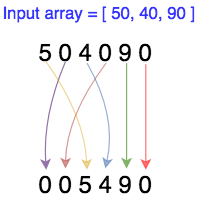## Algorithm

Problem Name: Mathematics - Constructing a Number

In this HackerRank in Mathematics - Constructing a Number solutions,

Manipulating numbers is at the core of a programmer's job. To test how well you know their properties, you are asked to solve the following problem.

You are given n non-negative integers a1,a2, .... , an . You want to know whether it's possible to construct a new integer using all the digits of these numbers such that it would be divisible by 3 . You can reorder the digits as you want. The resulting number can contain leading zeros.

For example, consider the numbers 50,40,90 from which you have to construct a new integer as described above. Numerous arrangements of digits are possible; but we have illustrated one below.Complete the function canConstruct which takes an integer array as input and return "Yes" or "No" based on whether or not the required integer can be formed.

Input Format

The first line contains a single integer t denoting the number of queries. The following lines describe the queries. Each query is described in two lines. The first of these lines contains a single integer n. The second contains n space-separated integers a1,a2, .... , an .

Constraints

• 1 <= t <= 1000
• 1 <= n <= 1000
• 1 <= ai <= 103

For 33.33% of the total score:

• n = 1
• 1 <= a1 <= 106

Output Format

For each query, print a single line containing "Yes" if it's possible to construct such integer and "No" otherwise.

Sample Input 0

3
1
9
3
40 50 90
2
1 4


Sample Output 0

Yes
Yes
No


## Code Examples

### #1 Code Example with C Programming

Code - C Programming


#include <stdio.h>
int main()
{
unsigned long long int r,c;
scanf("%llu%llu",&r,&c);
if(r%2 == 0)
{
unsigned long long int term = ((r-1)/2)*5+c;
printf("%llu",2*term-1); // nth term in odd series = 2*n-1
}
else{
unsigned long long int term = ((r)/2)*5+c;
printf("%llu", 2*term-2); // nth term in even series = 2*n-2
}
return 0;
}


Copy The Code &

### #2 Code Example with C++ Programming

Code - C++ Programming


#include <cstdio>
using namespace std;

int main() {
while(getchar_unlocked() > '\n')
{}
int c = 0;
while(c >= 0) {
while(getchar_unlocked() > '\n')
{}
int n = 0;
while((c = getchar_unlocked()) > '\n') {
if(c >= '0')
n += c;
}
puts(n % 3 ? "No" : "Yes");
}
return 0;
}


Copy The Code &